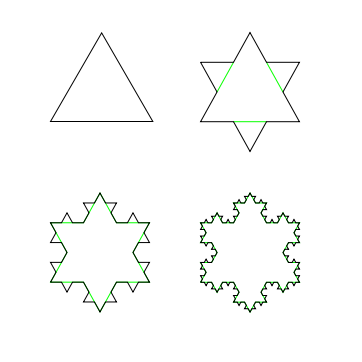# The Ultimate SnowflakeAn ultimate snowflake (Koch Snowflake) is formed when each side of an equilateral triangle is divided in $3$ equal parts, and a new equilateral triangle is constructed in the middle part. Repeat this step infinitely for each side of the polygon formed. Given that the side of the initial equilateral triangle of one such ultimate snowflake is 5 units, find the square of the area of the polygon (after repeating the above process infinitely).

×